# Calculating the Times Interest Earned Ratio For the most recent year

Calculating the Times Interest Earned Ratio For the most recent year, ICU Windows, Inc., had sales of \$380,000, cost of goods sold of \$110,000, depreciation expense of \$32,000, and additions to retained earnings of \$41,620. The firm currently has 30,000 shares of common stock outstanding, and the previous year's dividends per share were \$1.50. Assuming a 34 percent income tax rate, what was the times interest earned ratio?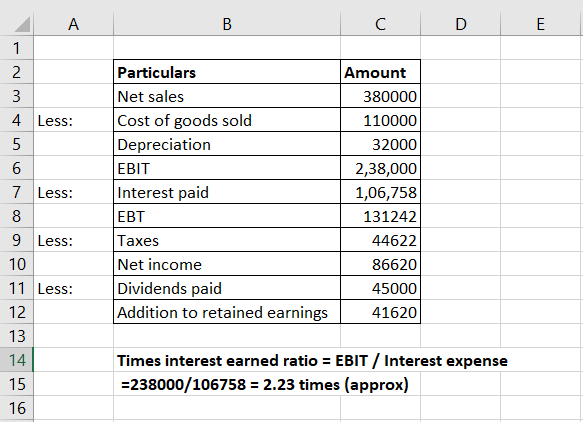Excel formula: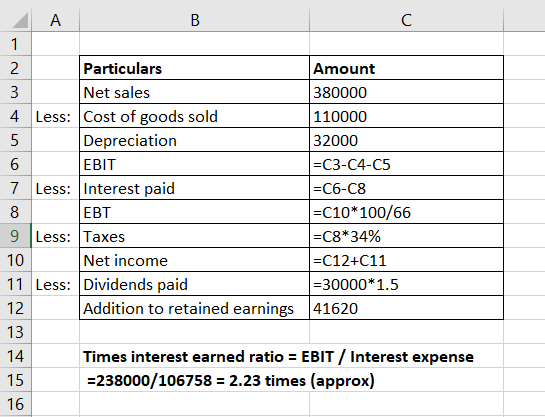Net Income =Addition to retained earnings+Dividend

= 41620+30000*1.5

= 41620+45000

= 86620

Net Income = (Sales- COGS-Depreciation-Interest)*(1-Tax rate)

86620= (380000-110000-32000-Interest)*(1-0.34)

131242.42= 238000-Interest

Interest = 106757.58

Times Interest Earned ratio = Interest/net Income = 106757.58/86620 = 1.23 times

EBIT = Sales – Costs - Depreciation expense = \$380,000 - \$110,000 - \$32,000 = \$238,000 Dividend = common stock outstanding × previous year’s dividends per share = \$30,000 × \$1.50 = \$45,000 Addition to Retained Earnings = Net Income – Dividend => Net Income = Addition to Retained Earnings + Dividend Net Income = \$41,620 + \$45,000 = \$86,620 Net Income = Earnings before Taxes × (1 – Tax Rate) => Earnings before Taxes = Net Income / (1 – Tax Rate) = \$86,620 / (1 – 0.34) = \$131,242.42 Taxes = Earnings before Taxes × Tax Rate = \$131,242.42 × 0.34 = \$44,622.42 Interest Expense = EBIT - Earnings before Taxes = \$238,000 - \$131,242.42 = \$106,757.58 Times Interest Earned = EBIT / Interest Expense = \$238,000 / \$106,757.58 = 2.22 times Times Interest Earned ratio = 2.22 times

#### Earn Coin

Coins can be redeemed for fabulous gifts.

Similar Homework Help Questions
• ### For the most recent year, Camargo, Inc., had sales of \$546,000, cost of goods sold of...

For the most recent year, Camargo, Inc., had sales of \$ 546,000, cost of goods sold of \$ 244,410, depreciation expense of \$ 61,900, and additions to retained earnings of \$ 74,300. The firm currently has 21,500 shares of common stock outstanding and the previous year's dividends per share were \$ 1.25.Assuming a 23 percent income tax rate, what was the times interest earned ratio? (Do not round intermediate calculations and round your answer to 2 decimal places, e.g., 32.16.)Times...

• ### 2.8 Mia, Inc. has a 19 percent ROA and a 20 percent payout ratio. a. (5...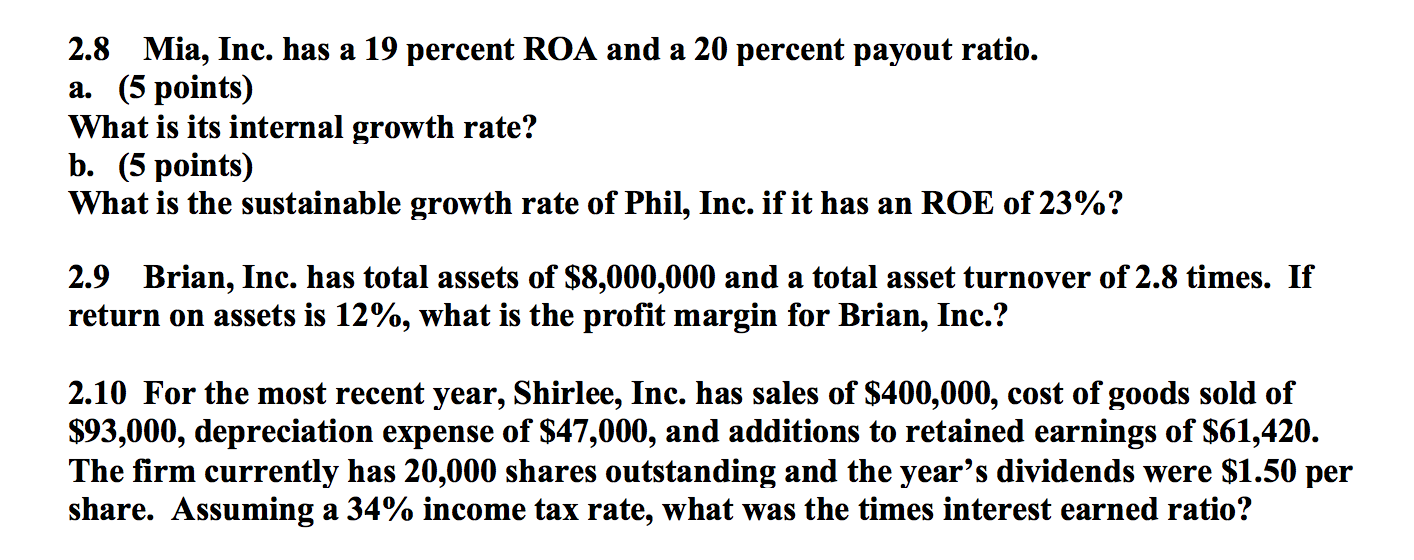2.8 Mia, Inc. has a 19 percent ROA and a 20 percent payout ratio. a. (5 points) What is its internal growth rate? b. (5 points) What is the sustainable growth rate of Phil, Inc. if it has an ROE of 23%? 2.9 Brian, Inc. has total assets of \$8,000,000 and a total asset turnover of 2.8 times. If return on assets is 12%, what is the profit margin for Brian, Inc.? 2.10 For the most recent year, Shirlee, Inc....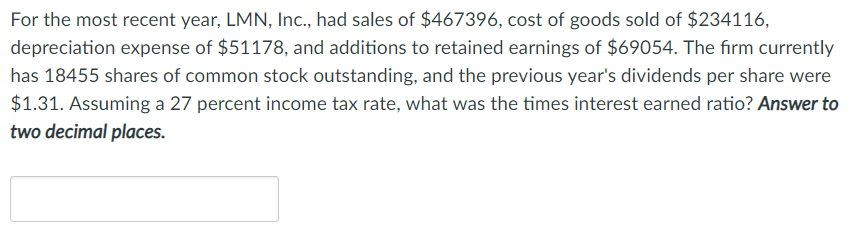Please make sure to double check your work for the correct answer & please show all steps! Thank you. For the most recent year, LMN, Inc., had sales of \$467396, cost of goods sold of \$234116, depreciation expense of \$51178, and additions to retained earnings of \$69054. The firm currently has 18455 shares of common stock outstanding, and the previous year's dividends per share were \$1.31. Assuming a 27 percent income tax rate, what was the times interest earned ratio?...

• ### finance 09

For the most recent year, Grohl, Inc., had sales of \$441,000, cost of goods sold of \$219,000, depreciation expense of \$58,700, and additions to retained earnings of\$50,900. The firm currently has 26,000 shares of common stock outstanding, and the previous year’s dividends per share were \$1.30Assuming a 35 percent income tax rate, what was the times interest earned ratio? (Round your answer to 2 decimal places (e.g., 32.16).)

• ### For the most recent year, Seether, Inc., had sales of \$439,000, cost of goods sold of...

For the most recent year, Seether, Inc., had sales of \$439,000, cost of goods sold of \$219,200, depreciation expense of \$58,900, and additions to retained earnings of \$51,100. The firm currently has 24,000 shares of common stock outstanding, and the previous year’s dividends per share were \$1.45. The income tax rate is 32 percent. What was the EBIT? (Do not round intermediate calculations and round your answer to the nearest whole number, e.g., 32.) EBIT            \$ How much in total...

• ### For the most recent year, Seether, Inc, had sales of \$454,000, cost of goods sold of...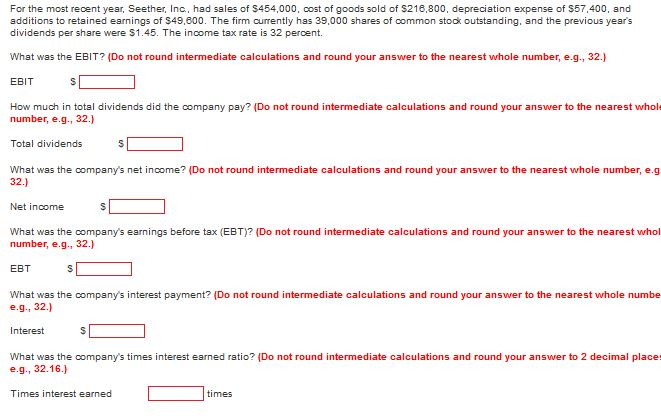For the most recent year, Seether, Inc, had sales of \$454,000, cost of goods sold of S218,800, depreciation expense of S57,400, and additions to retained earnings of \$49,800. The firm currently has 39,000 shares of common stock outstanding, and the previous years dividends per share were S1.45. The income tax rate is 32 percent. What was the EBIT? (Do not round intermediate calculations and round your answer to the nearest whole number, e.g., 32.) EBIT How much in total dividends...

• ### for the most recent year Seether, inc had sales of 449000 connect Chapter 3 Homework Instructions...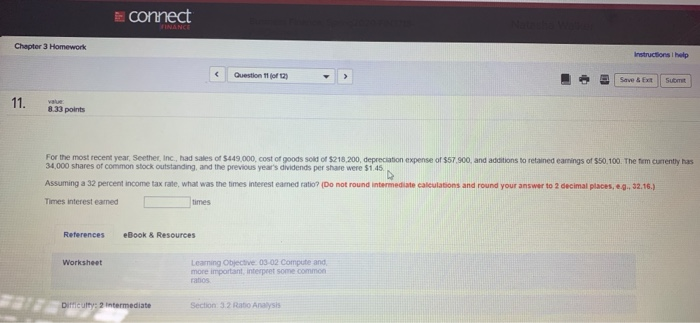for the most recent year Seether, inc had sales of 449000 connect Chapter 3 Homework Instructions help • Save & Submit 11. 33 points For the most recent year. Seether Inc had sales of \$449000, cost of goods some of \$218.200 depreciation expense of \$57.900, and additions to retained earnings of \$50.100. The fem currently has 34.000 shares of common stock outstanding and the previous year's dividends per share were \$1.45 Assuming a 32 percent income tax rate what was...

• ### 8. Ratio of Liabilities to Stockholders' Equity and Number of Times Interest Earned The following data...

8. Ratio of Liabilities to Stockholders' Equity and Number of Times Interest Earned The following data were taken from the financial statements of Hunter Inc. for December 31 of two recent years: Current Year Previous Year Accounts payable \$150,000 \$235,000 Current maturities of serial bonds payable 310,000 310,000 Serial bonds payable, 10% 1,630,000 1,940,000 Common stock, \$1 par value 80,000 110,000 Paid-in capital in excess of par 920,000 920,000 Retained earnings 3,180,000 2,520,000 The income before income tax was \$679,000...

• ### XYZ Ltd is attempting to calculate some of their ratios for their most recent fiscal year....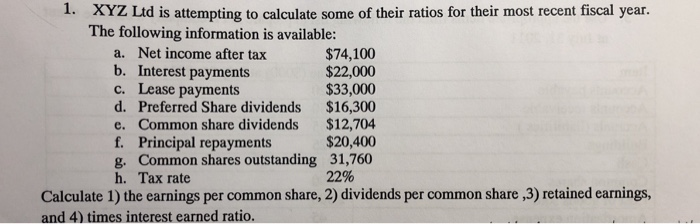XYZ Ltd is attempting to calculate some of their ratios for their most recent fiscal year. The following information is available: 1. a. Net income after tax b. Interest payments c. Lease payments d. Preferred Share dividends \$16,300 e. Common share dividends \$12,704 f. Principal repayments g. Common shares outstanding 31,760 h. Tax rate \$74,100 \$22,000 \$33,000 \$20,400 22% Calculate 1) the earnings per common share, 2) dividends per common share ,3) retained earnings, and 4) times interest earned ratio

• ### Ratio of Liabilities to Stockholders' Equity and Number of Times Interest Earned The following data were...

Ratio of Liabilities to Stockholders' Equity and Number of Times Interest Earned The following data were taken from the financial statements of Hunter Inc. for December 31 of two recent years: Current Year Previous Year Accounts payable \$340,000 \$101,000 Current maturities of serial bonds payable 230,000 230,000 Serial bonds payable, 10% 930,000 1,160,000 Common stock, \$1 par value 50,000 70,000 Paid-in capital in excess of par 550,000 550,000 Retained earnings 1,900,000 1,510,000 The income before income tax was \$359,600 and...